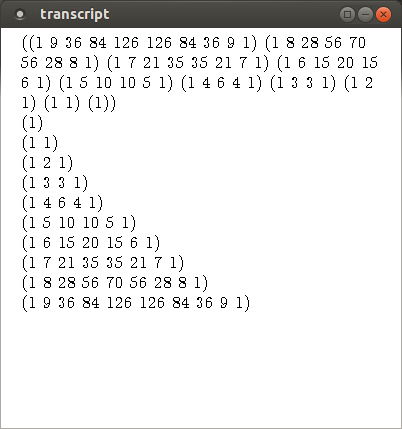# More Examples of Recursive Functions

## Recursive append

The recursive definition of append follows: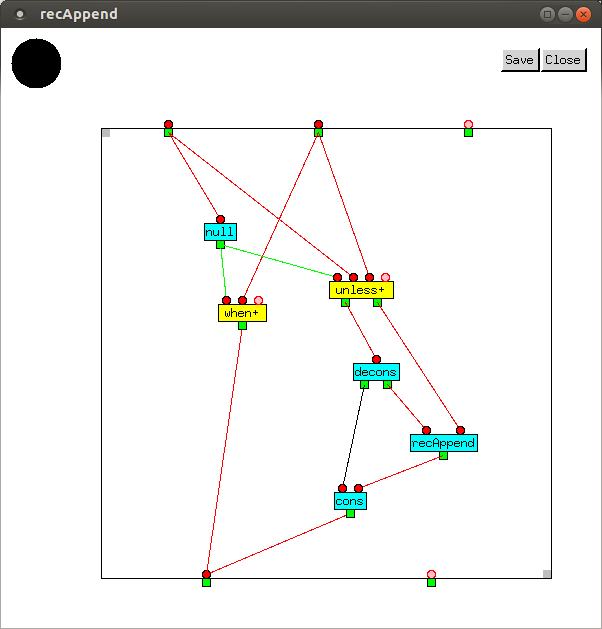It is equivalent to the Lisp

```(defun recAppend (x y)
(if (null x)
y
(cons (car x) (recAppend (cdr x) y))))
```

If the first argument is `NIL`, `recAppend` returns the second argument. Otherwise it joins the two lists together.

`decons` is short for deconstruct and takes a `List` and returns two values: its first element and the rest of the list. This does the work of the `(car x)` and `(cdr x)` calls in the `recAppend` Lisp function. If the inputs to `recAppend` are `(a b c)` and `(d e)`, the values produced at each stage are

 Inputs Vertex Outputs `NIL(a b c)` `unless+` `(a b c)` `(a b c)` `decons` `a(b c)` `(b c)(d e)` `recAppend` `(b c d e)` `a(b c d e)` `cons` `(a b c d e)`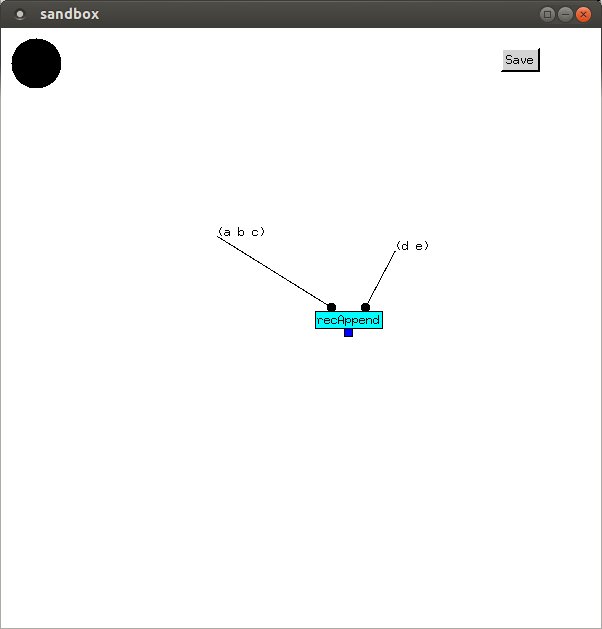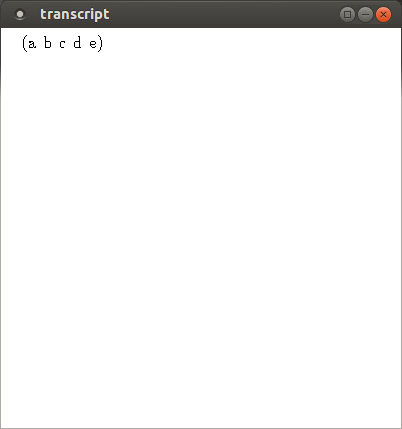## Tail-recursive reverse

`recAppend` is not the best implementation of `append`, as the repeated recursive calls require a lot of stack space. For very long lists this would result in stack overflow. It would be better to reverse the first argument, and then reverse it onto the second. This gives us the same answer, but in addition, gives us a reverse function. It would be best to implement reverse iteratively, which will be done in a later tutorial, but first we give a tail-recursive implementation.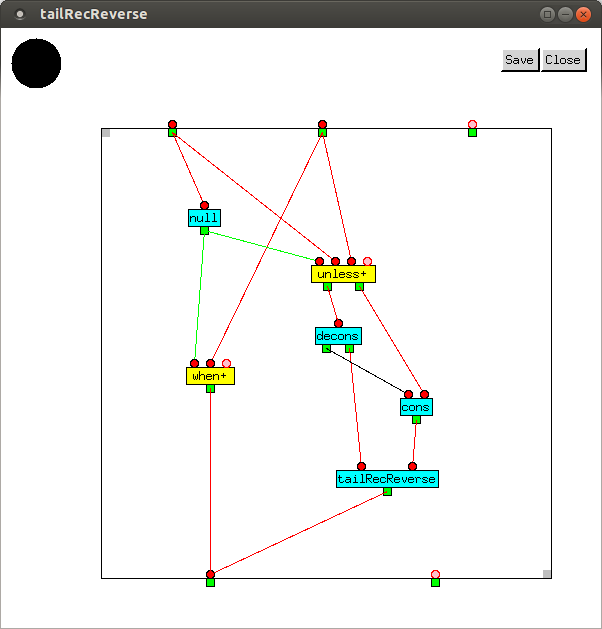It is equivalent to the Lisp

```(defun tailRecReverse (x y)
(if (null x)
y
(tailRecReverse (cdr x) (cons (car x) y))))
```

If the first argument is NIL, `tailRecReverse` returns the second argument. Otherwise, it reverses the first argument onto the second, e.g.
`(tailRecReverse '(c b a) '(d e))` returns `(a b c d e)`.

 Inputs Vertex Outputs `NIL(a b c)` `unless+` `(a b c)` `(a b c)` `decons` `a(b c)` `aNIL` `cons` `(a)` `(b c)(a)` `tailRecReverse` `(c b a)`

In the sandbox, first `tailRecReverse` is called to reverse a list. Then, `tailRecReverse` is called twice, giving the same result we would get were `recAppend` called instead: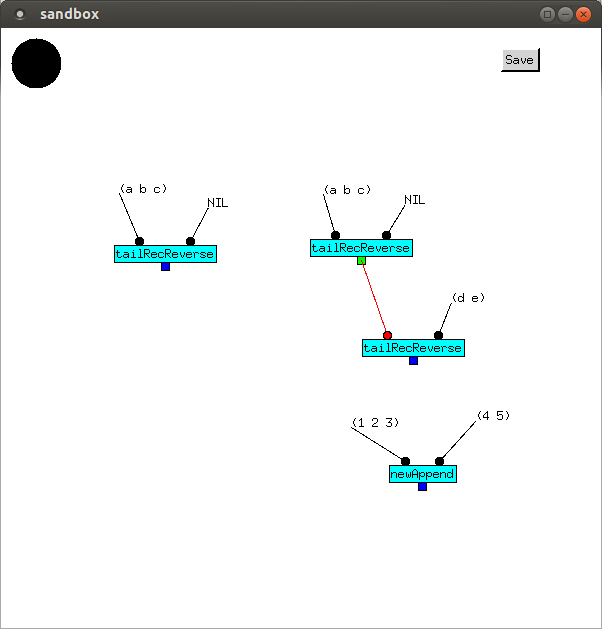We can wrap the two calls into a new append function, and then call that: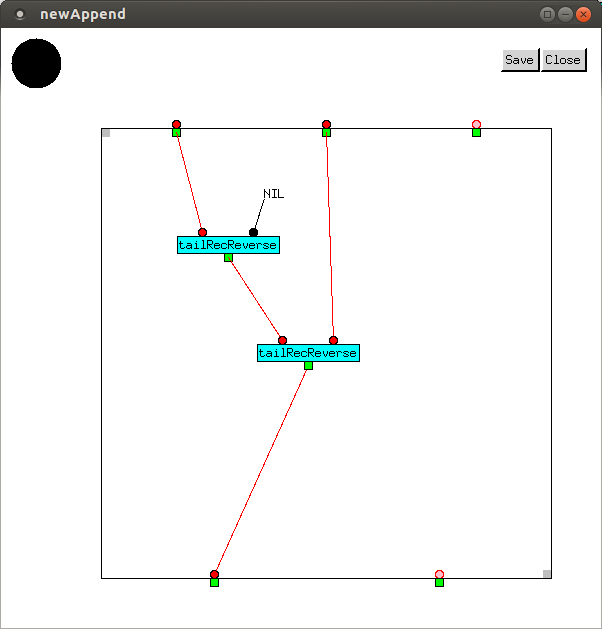The results of making the three calls are: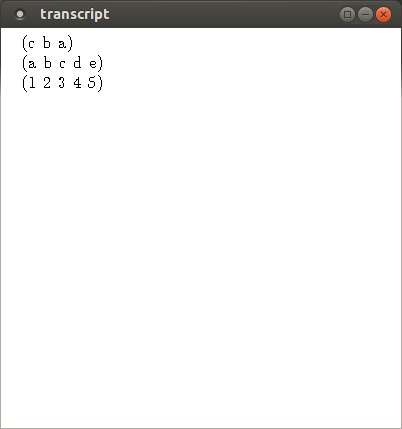## Pascal's triangle

Now, let's look at a more complex function, to compute Pascal's triangle.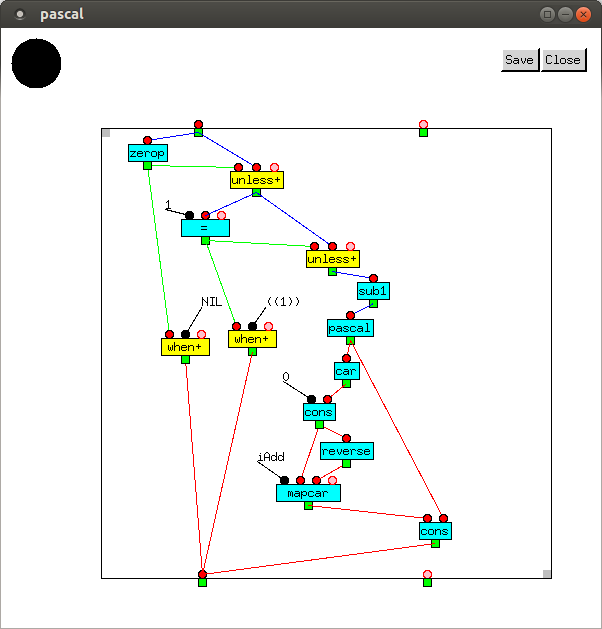This builds up a list of lists of integers in reverse order. The first two branches which output `NIL` when the input is `0`, and `((1))` when the input is 1, are the base cases. The other branch will be easier to understand by going through the values produced at each stage:

 Inputs Vertex Outputs `16` `=` `NIL` `NIL6` `unless+` `6` `6` `sub1` `5` `5` `pascal` `((1 4 6 4 1) (1 3 3 1) (1 2 1) (1 1) (1))` `((1 4 6 4 1) (1 3 3 1) (1 2 1) (1 1) (1))` `car` `(1 4 6 4 1)` `0 (1 4 6 4 1)` `cons` `(0 1 4 6 4 1)` `(0 1 4 6 4 1)` `reverse` `(1 4 6 4 1 0)` `iAdd (0 1 4 6 4 1) (1 4 6 4 1 0)` `mapcar` `(1 5 10 10 5 1)` `(1 5 10 10 5 1)((1 4 6 4 1) (1 3 3 1) (1 2 1) (1 1) (1))` `cons` `((1 5 10 10 5 1) (1 4 6 4 1) (1 3 3 1) (1 2 1) (1 1) (1))`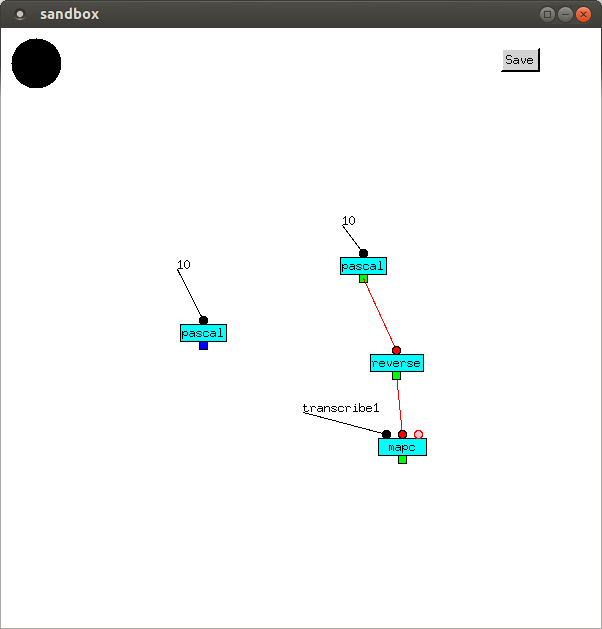The first attempt at output wasn't particularly readable, so we reversed the output of `pascal` to get it in the usual order, and then transcribed each list separately. This was done by mapping `transcribe1` (which transcribes a single value) over each list comprising `pascal`'s output.